# Electric potential

Table of Content

## Electric Potential

• We now move towards the electric potential which is potential energy per unit charge.
• Thus electrostatic potential at any point of an electric field is defined as potential energy per unit charge at that point.
• Electric potential is represented by letter V.
V=U/q' or U=q'V ----(6)
• Electric potential is a scalar quantity since both charge and potential energy are scalar quantities.
• S.I. unit of electric potential is Volt which is equal to Joule per Coulomb. Thus,
1 Volt = 1 JC-1
• In equation 4 if we divide both sides by q' we have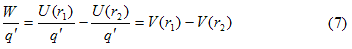where V(r1) is the potential energy per unit charge at point R and V(r2) is potential energy per unit charge at point S and are known as potential at points R and S respectively.
• Again consider figure 1. If point S in figure 1 would be at infinity then from equation 7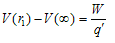Since potential energy at infinity is zero therefore V(∞)=0. Thereforehence electric potential at a point in an electric field is the ratio of work done in bringing test charge from infinity to that point to the magnitude of test charge.
• Dimensions of electric potential are [ML2T-3A-1] and can be calculated easily using the concepts of dimension analysis.

## Electric potential due to a point charge

• Consider a positive test charge +q is placed at point O shown below in the figure.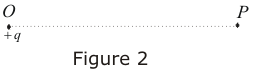• We have to find the electric potential at point P at a distance r from point O.
• If we move a positive test charge q' from infinity to point P then change in electric potential energy would be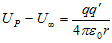• Electric potential at point P is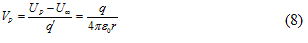• here we see that like electric field potential at any point independent of test charge used to define it.
• Here are the graph of potential with respect to distance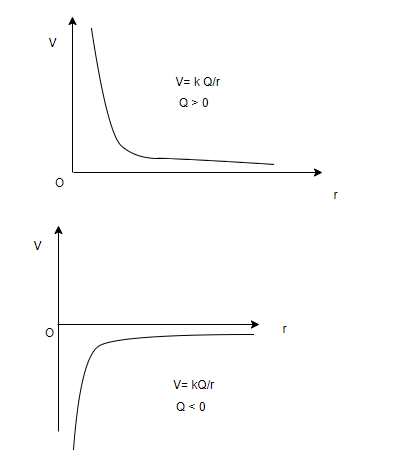## Electric Potential due to a system of charges

• Consider a system of charges $q_1$, $q_2$,...., $q_n$ with position vectors $\mathbf{r_1}$,$\mathbf{r_2}$,..., $\mathbf{r_n}$ relative to some origin
• Potential at any point will be the sum of potential of the individual charges $V=V_1 + V_2 ... + V_n$
• Potential V at any point due to arbitrary collection of point charges is given by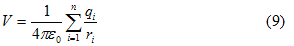• For continuous charge distributions summation in above expression will be replaced by the integration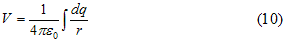where dq is the differential element of charge distribution and r is its distance from the point at which V is to be calculated.

Question -1
(a)Calculate the potential at a point P due to a charge of $1 \times 10^{-7} \ C$ located 9 cm away.
(b) Hence obtain the work done in bringing a charge of $1 \times 10^{-9} \ C$ from infinity to the point P. Does the answer depend on the path along which the charge is brought?
Given $\frac {1}{4 \pi \epsilon _0}=9 \times 10^9 \ Nm^2/C^2$
Solution
(a) Potential is given by the formula
$V= \frac {1}{4 \pi \epsilon _0} \frac {Q}{r}$
Here Q=$1 \times 10^{-7} \ C$ and $r=.09 \ m$
$V= 9 \times 10^9 \times \frac {1 \times 10^{-7}}{.09}=10^4 \ V$
(b)Work done is given by
$W= qV= 1 \times 10^{-9} \times 10^4= 10^{-5} \ J$
Work done will be path independent